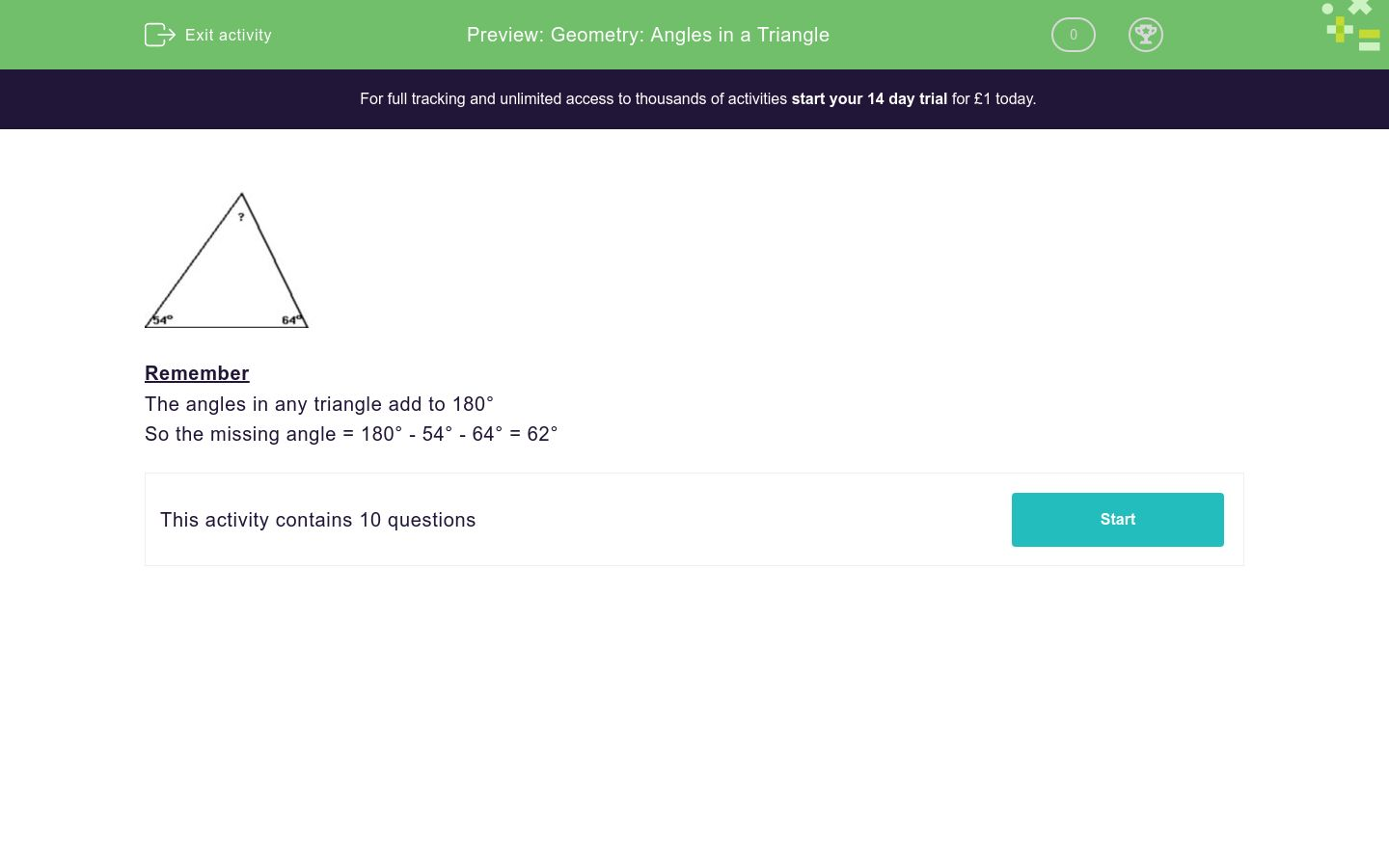# Geometry: Angles in a Triangle

This worksheet is about calculating the third angle in a triangle.Key stage:  KS 2

Curriculum topic:   Geometry: Properties of Shapes

Curriculum subtopic:   Identify Angles at a Point

Difficulty level:### QUESTION 1 of 10Remember

The angles in any triangle add to 180°

So the missing angle = 180° - 54° - 64° = 62°

Two of the angles in a triangle are 77º and  66º.

Work out the size of the third angle in degrees.

(Just write the number)

Two of the angles in a triangle are 75º and  65º.

Work out the size of the third angle in degrees.

(Just write the number)

Two of the angles in a triangle are 68º and  67º.

Work out the size of the third angle in degrees.

(Just write the number)

Two of the angles in a triangle are 25º and  42º.

Work out the size of the third angle in degrees.

(Just write the number)

Two of the angles in a triangle are 25º and  25º.

Work out the size of the third angle in degrees.

(Just write the number)

Two of the angles in a triangle are 130º and  25º.

Work out the size of the third angle in degrees.

(Just write the number)

Two of the angles in a triangle are 101º and  25º.

Work out the size of the third angle in degrees.

(Just write the number)

Two of the angles in a triangle are 131º and  25º.

Work out the size of the third angle in degrees.

(Just write the number)

Two of the angles in a triangle are 97º and  36º.

Work out the size of the third angle in degrees.

(Just write the number)

Two of the angles in a triangle are 170º and  .

Work out the size of the third angle in degrees.

(Just write the number)

• Question 1

Two of the angles in a triangle are 77º and  66º.

Work out the size of the third angle in degrees.

(Just write the number)

37
EDDIE SAYS
180 - 77 - 66 = 37
• Question 2

Two of the angles in a triangle are 75º and  65º.

Work out the size of the third angle in degrees.

(Just write the number)

40
EDDIE SAYS
180 - 75 - 65 = 40
• Question 3

Two of the angles in a triangle are 68º and  67º.

Work out the size of the third angle in degrees.

(Just write the number)

45
EDDIE SAYS
180 - 68 - 67 = 45
• Question 4

Two of the angles in a triangle are 25º and  42º.

Work out the size of the third angle in degrees.

(Just write the number)

113
EDDIE SAYS
180 - 25 - 42 = 113
• Question 5

Two of the angles in a triangle are 25º and  25º.

Work out the size of the third angle in degrees.

(Just write the number)

130
EDDIE SAYS
180 - 25 - 25 = 130
• Question 6

Two of the angles in a triangle are 130º and  25º.

Work out the size of the third angle in degrees.

(Just write the number)

25
EDDIE SAYS
180 - 130 - 25 = 25
• Question 7

Two of the angles in a triangle are 101º and  25º.

Work out the size of the third angle in degrees.

(Just write the number)

54
EDDIE SAYS
180 - 101 - 25 =
• Question 8

Two of the angles in a triangle are 131º and  25º.

Work out the size of the third angle in degrees.

(Just write the number)

24
EDDIE SAYS
180 - 131 - 25 = 24
• Question 9

Two of the angles in a triangle are 97º and  36º.

Work out the size of the third angle in degrees.

(Just write the number)

47
EDDIE SAYS
180 - 97 - 36 = 47
• Question 10

Two of the angles in a triangle are 170º and  .

Work out the size of the third angle in degrees.

(Just write the number)

1
EDDIE SAYS
180 - 170 - 9 = 1
---- OR ----

Sign up for a £1 trial so you can track and measure your child's progress on this activity.

### What is EdPlace?

We're your National Curriculum aligned online education content provider helping each child succeed in English, maths and science from year 1 to GCSE. With an EdPlace account you’ll be able to track and measure progress, helping each child achieve their best. We build confidence and attainment by personalising each child’s learning at a level that suits them.

Get started